# Calculator sum mixed number and an improper fraction

The calculator performs basic and advanced operations with mixed numbers, fractions, integers, decimals. Mixed fractions are also called mixed numbers. A mixed fraction is a whole number and a proper fraction combined, i.e. one and three-quarters. The calculator evaluates the expression or solves the equation with step-by-step calculation progress information. Solve problems with two or more mixed numbers fractions in one expression.

### 13/5 + 11/5 = 19/5 = 3 4/5 = 3.8

Spelled result in words is three and four fifths (or nineteen fifths).

### Calculation steps

1. Conversion a mixed number 1 3/5 to a improper fraction: 1 3/5 = 1 3/5 = 1 · 5 + 3/5 = 5 + 3/5 = 8/5

To find new numerator:
a) Multiply the whole number 1 by the denominator 5. Whole number 1 equally 1 * 5/5 = 5/5
b) Add the answer from previous step 5 to the numerator 3. New numerator is 5 + 3 = 8
c) Write a previous answer (new numerator 8) over the denominator 5.

One and three fifths is eight fifths
2. Add: 8/5 + 11/5 = 8 + 11/5 = 19/5
For adding, subtracting, and comparing fractions, it is suitable to adjust both fractions to a common (equal, identical) denominator. The common denominator you can calculate as the least common multiple of both denominators - LCM(5, 5) = 5. In practice, it is enough to find the common denominator (not necessarily the lowest) by multiplying the denominators: 5 × 5 = 25. In the next intermediate step, the fraction result cannot be further simplified by canceling.
In words - eight fifths plus eleven fifths = nineteen fifths.

## Mixed fractions in word problems:

• A science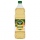A science experiment calls for making 3 and 2/3 cups of distilled water with 1 and 3/5 cups of vinegar and 2/3 cups of liquid detergent how much liquid in all and cups is needed?Add this two mixed numbers: 1 5/6 + 2 2/11=3 3/4 + 2 3/5 + 5 1/2 Show your solution.2 and 1/8th plus 1 and 1/3rd =
• A full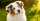A full bag of dog food weights 5 1/2 pounds. How much dog food do you have if there are 3 3/4 bags in the cupboard?Add two mixed fractions: 2 4/6 + 1 3/6
• Donuts 3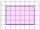You pick up donuts for a party. You have 3 and 4/12 boxes of donuts. Roy picks up donuts too. He has 2 and 9/12 boxes of donuts. How many boxes of donuts do you have in all?
• Sum of mixedThe sum of two mixed numbers is 15½. One of the number is 5 ¾, what is the other?
• Jack planted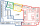Jack planted 1 1/4 rows of lettuce in the family garden, Rose planted 1 1/2 rows of broccoli and Calvin planted 4 5/12 rows of beans. How many rows were planted in the garden?
• Sum of 20Sum of the two numbers is 15 1/6. If one of the numbers is 2 2/3, find the other number.Add this two mixed numbers: 1 5/6 + 2 2/11=The first team member in a 926-person relay race must run 2 1/4 laps, the second team member must run 1 1/2 laps, and the third team member must run 3 1/4 laps. How many laps in all must each team run?Sum of two fractions is 4 3/7. If one of the fractions is 2 1/5 find the other one .Ananya has a bunny. She bought 4 7/8 pounds of carrots. She fed her bunny 1 1/4 pounds of carrots the first week. She fed her bunny 5/6 pounds of carrots the second week. All together, how many pounds of carrots did she feed her bunny? 1. Draw a tape diag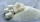Luana is making a recipe that uses 2 7/8 cups of sugar. She has 1 1/4 cups of sugar in her sugar bowl and 7 1/2 cups of sugar in the canister. How many cups of sugar will Luana have left after she makes her recipe?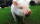In the morning, Christopher puts out 7 3/5 pounds of food for the piglets on his farm. In the evening, he puts out another 6 4/5 pounds. How much food does Christopher put out in all?What is 4 1/2+2/7-213/14?